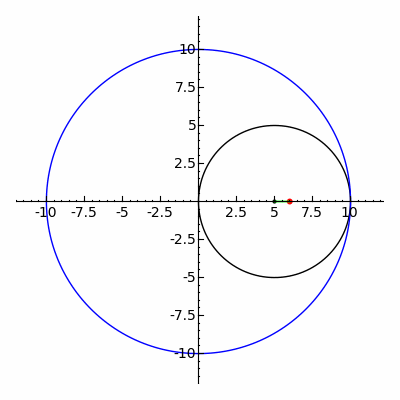# 离散傅里叶变换一则：中点多边形谜题(1) 自身相交的多边形，取其多次中点多边形之后是否一定会自身不相交？
(2) 自身不相交的多边形，取其多次中点多边形之后是否一定变为凸多边形？
(3) 一个凸多边形，取其多次中点多边形之后是否趋于正多边形？

(^o^)っ

(3) 是否定的。一个很扁的长方形会变成一个很扁的菱形，然后变回原始的长方形的相似形。

（既然碰巧说到了就顺带一提，任意四边形的中点四边形都是个平行四边形，所以 “中点多边形可以取到任意形状” 的这个命题也并不成立。）

(1) 是否定的。一个正五角星的中点多边形是一个正五角星。

(2) 也是否定的。请看下面的构造：Berlekamp-Gilbert-Sinden 定理 (1965)： 对几乎所有的多边形，前面的命题 (1) 和 (2) 无条件成立，中点多边形将无限趋近于一个“椭圆”的正多边形，且有奇数条边的多边形总是某个其他多边形的中点多边形。$x_i=\sum_{c=0}^{n-1}a_c\omega_n^{ci}\tag{\dagger}$

$\underbrace{\frac1n\sum_{i=0}^{n-1}}_{求重心}\left(x_i\omega_n^{-si}\right)=\underbrace{a_s}_{定点}\tag{\ddagger}$

s可以取任意数，所以这实际上就是我们想要的正n角星 $$a_s\omega_n^{si}$$ 的表达了。我们现在只剩下两件事：验证 $$x_i$$ 确实是这些正n角星的和，以及之前欠下的 $$a_0=0$$。验证这个和与之前求 $$a_s$$ 的方法几乎是一样的：

\begin{align*} \sum_{s=0}^{n-1}a_s\omega_n^{si}&=\sum_{s=0}^{n-1}\left(\frac{1}{n}\sum_{j=0}^{n-1}x_j\omega_n^{-sj}\right)\omega_n^{si}\\ &=\sum_{j=0}^{n-1}\underbrace{\left(\frac{1}{n}\sum_{s=0}^{n-1}x_j\omega_n^{(i-j)s}\right)}_{正n角星的重心}\\ &=\sum_{j=0}^{n-1} \begin{cases} 0&(j\ne i)\\ x_i&(j=i) \end{cases}\\ &=x_i \end{align*}

$a_0=\underbrace{\frac{1}{n}\sum_{i=0}^{n-1}x_i}_{x的重心}=0$

$t_c=\left|\cos\frac{c\pi}n\right|$